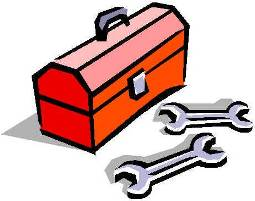# R Helper FunctionsIf you do a lot of R programming, you probably have a list of R helper functions set aside in a script that you include on R startup or at the top of your code. In some cases helper functions add capabilities that aren’t otherwise available. In other cases, they replicate functionality that is available elsewhere without loading unnecessary components. Below I present two of my most frequently used data manipulation helper functions as examples.

### Descriptives R Helpler Function

``````# Display some basic descriptives
descs <- function (x) { if(!hidetables) { if(length(unique(x))>30) {
print("Summary results:")
print(summary(x))
print("")
print("Number of categories is greater than 30, table not produced")
} else {
print("Summary results:")
print(summary(x))
print("")
print("Table results:")
print(table(x, useNA="always"))
}

} else {
print("Tables are hidden")
}
}

# Set hide tables to true to hide tables``````

### Dummy Variable R Helper Function

``````# Create dummy variables for each level of a categorical variable
createDummies <- function(x, df, keepNAs = TRUE) { for (i in seq(1, length(unique(df[, x])))) { if(keepNAs) { df[, paste(x,".", i, sep = "")] <- ifelse(df[, x] != i, 0, 1) } else { df[, paste(x,".", i, sep = "")] M- ifelse(df[, x] != i | is.na(df[, x]) , 0, 1) } } df } ``````

The Descriptives R Helper Function produces a summary or table of the passed variable/object; it uses the number of unique values to determine whether to call just the summary() or summary() and table() functions. It also includes NAs by default in the tables (one of `table()`‘s biggest annoyances). Once the exploratory and data manipulation work is done, all output from this function can be suppressed by setting the `hidetables` object to TRUE.

The Dummy Variable R Helper Function creates indicator variables from all values of a variable. Based on experience, I avoid the factor object as much as possible and this approach allows me to quickly create indicators that can be used in any way I want.

If you don’t have a programming background or are just beginning with R, you might not have had time to realize the benefit of helper functions or identify the tasks you do repetitively, but it’s worthwhile to give the issue some consideration. Helper functions can be exceptionally useful for saving time on repetitive tasks or facilitating your work. They’re so useful in fact, that there is a special ProgrammingR event planned around helper functions coming soon. For the more experienced R programmers out there, make a mental note of the most useful helper functions you’ve written in the past. That list will come in handy in the near future!

R Helper Functions
Scroll to top